## RS Aggarwal Class 10 Solutions Chapter 2 Polynomials Test Yourself

These Solutions are part of RS Aggarwal Solutions Class 10. Here we have given RS Aggarwal Class 10 Solutions Chapter 2 Polynomials Test Yourself

Other Exercises

MCQ
Question 1.
Solution:
(b) Polynomial is x2 – 2x – 3
=> x2 – 3x + x – 3
= x(x – 3) + 1(x – 3)
= (x – 3) (x + 1)
Either x – 3 = 0, then x = 3
or x + 1 = 0, then x = -1
Zeros are 3, -1

Question 2.
Solution:
(a) α, β, γ are the zeros of Polynomial is x3 – 6x2 – x + 30
Here, a = 1, b = -6, c = -1, d = 30
αβ + βγ + γα = $$\frac { c }{ a }$$ = $$\frac { -1 }{ 1 }$$ = -1

Question 3.
Solution:
(c)Question 4.
Solution:
(c) Let α and β be the zeros of the polynomial 4x2 – 8kx + 9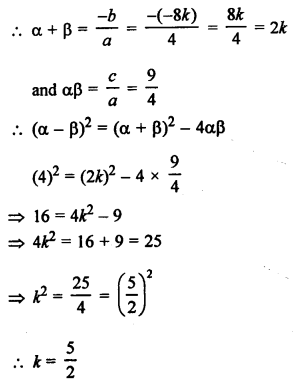Question 5.
Solution: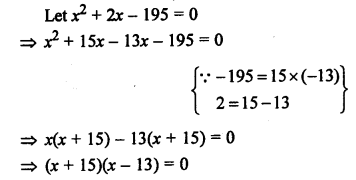Either x + 15 = 0, then x = -15
or x – 13 = 0, then x = 13
Zeros are 13, -15

Question 6.
Solution:
The polynomial is (a2 + 9) x2 + 13x + 6a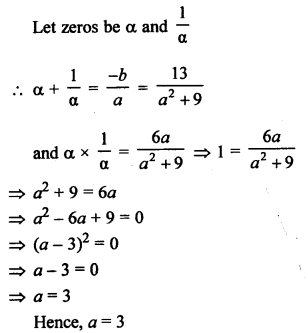Question 7.
Solution:
Zeros are 2 and -5
Sum of zeros = 2 + (-5) = 2 – 5 = -3
and product of zeros = 2 x (-5) = -10
Now polynomial will be
x2 – (Sum of zeros) x + Product of zeros
= x2 – (-3)x + (-10)
= x2 + 3x – 10

Question 8.
Solution:
(a – b), a, (a + b) are the zeros of the polynomial x3 – 3x2 + x + 1
Here, a = 1, b = -3, c = 1, d = 1
Now, sum of zeros = $$\frac { -b }{ a }$$ = $$\frac { -(-3) }{ 1 }$$ = 3
a – b + a + a + b = 3Question 9.
Solution:
Let f(x) = x3 + 4x2 – 3x – 18
If 2 is its zero, then it will satisfy it
Now, (x – 2) is a factor Dividing by (x – 2)
Hence, x = 2 is a zero of f(x)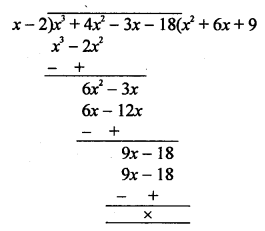Question 10.
Solution:
Sum of zeros = -5
and product of zeros = 6
x2 – (Sum of zeros) x + Product of zeros
= x2 – (-5) x + 6
= x2 + 5x + 6

Question 11.
Solution: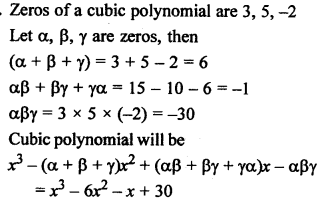Question 12.
Solution:
p(x) = x3 + 3x2 – 5x + 4
g(x) = x – 2
Let x – 2 = 0, then x = 2
Remainder = p(2) = (2)3 + 3(2)2 – 5(2) + 4 = 8 + 12 – 10 + 4 = 14

Question 13.
Solution:
f(x) = x3 + 4x2 + x – 6
and g(x) = x + 2
Let x + 2 = 0, then x = -2
f(-2) = (-2)3 + 4(-2)2 + (-2) – 6 = -8 + 16 – 2 – 6 = 0
Remainder is zero, x + 2 is a factor of f(x)

Question 14.
Solution: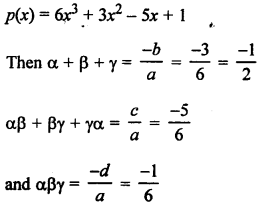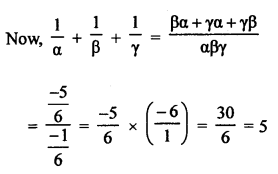Question 15.
Solution: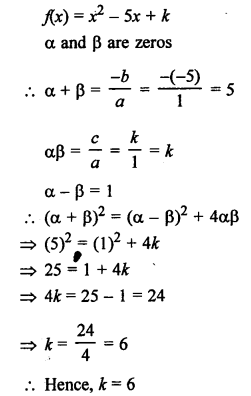Question 16.
Solution:
f(x) = x4 + 4x2 + 6
=> (x2)2 + 4(x2) + 6 = y2 + 4y + 6 (Let x2 = y)
Let α, β be the zeros of y2 + 4y + 6
Sum of zeros = -4
and product of zeros = 6
But there are no factors of 6 whose sum is -4 {Factors of 6 = 1 x 6 and 2 x 3}
Hence, f(x) Has no zero (real).

Question 17.
Solution:
3 is one zero of p(x) = x3 – 6x2 + 11x – 6
(x – 3) is a factor of p(x)
Dividing, we getQuestion 18.
Solution: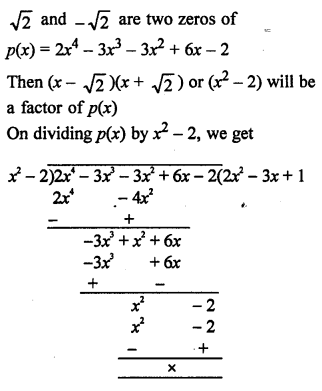Question 19.
Solution:
p(x) = 3x4 + 5x3 – 7x2 + 2x + 2
Dividing by x2 + 3x + 1,
we get,
Quotient = 3x2 – 4x + 2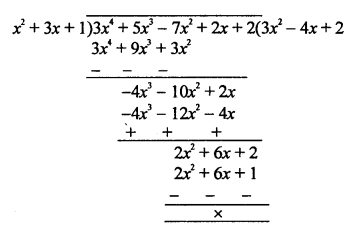Question 20.
Solution:
Let p(x) = x3 + 2x2 + kx + 3
g(x) = x – 3
and r(x) = 21
Dividing p(x) by g(x), we get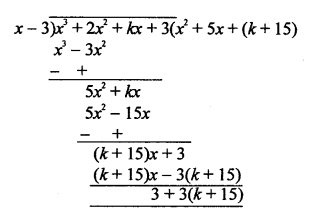But remainder = 21
3 + 3k + 45 = 21
3k = 21 – 45 – 3
=> 3k = 21 – 48 = -27
k = -9
Second method:
x – 3 is a factor of p(x) : x = 3
Substituting the value of x in p(x)
p(3) = 33 + 2 x 32 + k x 3 + 3
= 27 + 18 + 3k + 3
48 + 3k = 21
=> 3k = -48 + 21 = -27
k = -9
Hence, k = -9

Hope given RS Aggarwal Solutions Class 10 Chapter 2 Polynomials Test Yourself are helpful to complete your math homework.

If you have any doubts, please comment below. Learn Insta try to provide online math tutoring for you.